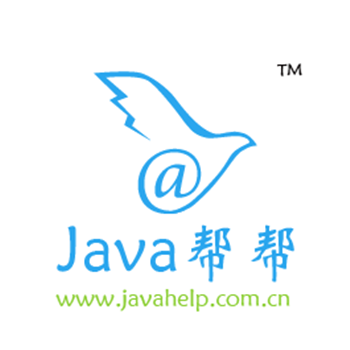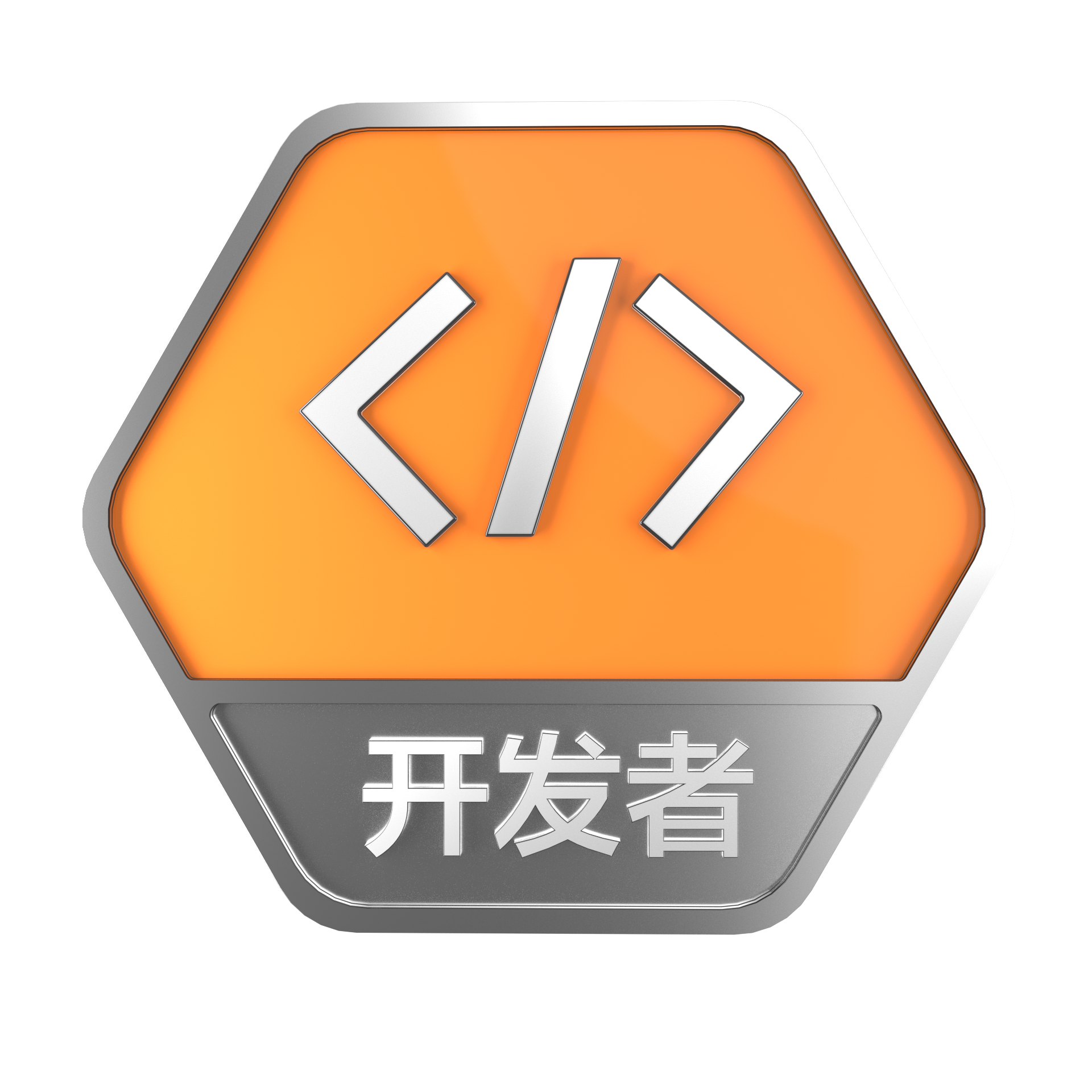# PostgreSQL 11 preview 分区过滤控制参数 - enable_partition_pruning

+关注继续查看

### 标签

PostgreSQL , 分区控制 , enable_partition_pruning

## 背景

PostgreSQL 10开始支持了分区表的语法，可以通过新的语法创建分区表，而更早的版本则需要使用inherit+check约束+rule/trigger来创建分区表。

《分区表锁粒度差异 - pg_pathman VS native partition table》

《PostgreSQL 传统 hash 分区方法和性能》

https://www.postgresql.org/docs/10/static/runtime-config-query.html#RUNTIME-CONFIG-QUERY-OTHER

PG 11增加了一个参数 enable_partition_pruning ，仅用于控制分区表（不用于控制inherit, union all等操作）的QUERY。

Add GUC enable_partition_pruning

This controls both plan-time and execution-time new-style partition
pruning.  While finer-grain control is possible (maybe using an enum GUC
instead of boolean), there doesn't seem to be much need for that.

This new parameter controls partition pruning for all queries:
trivially, SELECT queries that affect partitioned tables are naturally
under its control since they are using the new technology.  However,
while UPDATE/DELETE queries do not use the new code, we make the new GUC
control their behavior also (stealing control from
constraint_exclusion), because it is more natural, and it leads to a
more natural transition to the future in which those queries will also
use the new pruning code.

Constraint exclusion still controls pruning for regular inheritance
situations (those not involving partitioned tables).

Author: David Rowley
Review: Amit Langote, Ashutosh Bapat, Justin Pryzby, David G. Johnston
Discussion: https://postgr.es/m/CAKJS1f_0HwsxJG9m+nzU+CizxSdGtfe6iF_ykPYBiYft302DCw@mail.gmail.com


## test case

+--
+-- Ensure the enable_partition_prune GUC properly disables partition pruning.
+--
+create table pp_lp (a int, value int) partition by list (a);
+create table pp_lp1 partition of pp_lp for values in(1);
+create table pp_lp2 partition of pp_lp for values in(2);
+explain (costs off) select * from pp_lp where a = 1;
+        QUERY PLAN
+--------------------------
+ Append
+   ->  Seq Scan on pp_lp1
+         Filter: (a = 1)
+(3 rows)
+
+explain (costs off) update pp_lp set value = 10 where a = 1;
+        QUERY PLAN
+--------------------------
+ Update on pp_lp
+   Update on pp_lp1
+   ->  Seq Scan on pp_lp1
+         Filter: (a = 1)
+(4 rows)
+
+explain (costs off) delete from pp_lp where a = 1;
+        QUERY PLAN
+--------------------------
+ Delete on pp_lp
+   Delete on pp_lp1
+   ->  Seq Scan on pp_lp1
+         Filter: (a = 1)
+(4 rows)

+set enable_partition_pruning = off;
+set constraint_exclusion = 'partition'; -- this should not affect the result.
+explain (costs off) select * from pp_lp where a = 1;
+        QUERY PLAN
+--------------------------
+ Append
+   ->  Seq Scan on pp_lp1
+         Filter: (a = 1)
+   ->  Seq Scan on pp_lp2
+         Filter: (a = 1)
+(5 rows)
+
+explain (costs off) update pp_lp set value = 10 where a = 1;
+        QUERY PLAN
+--------------------------
+ Update on pp_lp
+   Update on pp_lp1
+   Update on pp_lp2
+   ->  Seq Scan on pp_lp1
+         Filter: (a = 1)
+   ->  Seq Scan on pp_lp2
+         Filter: (a = 1)
+(7 rows)
+
+explain (costs off) delete from pp_lp where a = 1;
+        QUERY PLAN
+--------------------------
+ Delete on pp_lp
+   Delete on pp_lp1
+   Delete on pp_lp2
+   ->  Seq Scan on pp_lp1
+         Filter: (a = 1)
+   ->  Seq Scan on pp_lp2
+         Filter: (a = 1)
+(7 rows)

+set constraint_exclusion = 'off'; -- this should not affect the result.
+explain (costs off) select * from pp_lp where a = 1;
+        QUERY PLAN
+--------------------------
+ Append
+   ->  Seq Scan on pp_lp1
+         Filter: (a = 1)
+   ->  Seq Scan on pp_lp2
+         Filter: (a = 1)
+(5 rows)
+
+explain (costs off) update pp_lp set value = 10 where a = 1;
+        QUERY PLAN
+--------------------------
+ Update on pp_lp
+   Update on pp_lp1
+   Update on pp_lp2
+   ->  Seq Scan on pp_lp1
+         Filter: (a = 1)
+   ->  Seq Scan on pp_lp2
+         Filter: (a = 1)
+(7 rows)
+
+explain (costs off) delete from pp_lp where a = 1;
+        QUERY PLAN
+--------------------------
+ Delete on pp_lp
+   Delete on pp_lp1
+   Delete on pp_lp2
+   ->  Seq Scan on pp_lp1
+         Filter: (a = 1)
+   ->  Seq Scan on pp_lp2
+         Filter: (a = 1)
+(7 rows)

+drop table pp_lp;
+-- Ensure enable_partition_prune does not affect non-partitioned tables.
+create table inh_lp (a int, value int);
+create table inh_lp1 (a int, value int, check(a = 1)) inherits (inh_lp);
+NOTICE:  merging column "a" with inherited definition
+NOTICE:  merging column "value" with inherited definition
+create table inh_lp2 (a int, value int, check(a = 2)) inherits (inh_lp);
+NOTICE:  merging column "a" with inherited definition
+NOTICE:  merging column "value" with inherited definition
+set constraint_exclusion = 'partition';
+-- inh_lp2 should be removed in the following 3 cases.
+explain (costs off) select * from inh_lp where a = 1;
+        QUERY PLAN
+---------------------------
+ Append
+   ->  Seq Scan on inh_lp
+         Filter: (a = 1)
+   ->  Seq Scan on inh_lp1
+         Filter: (a = 1)
+(5 rows)
+
+explain (costs off) update inh_lp set value = 10 where a = 1;
+        QUERY PLAN
+---------------------------
+ Update on inh_lp
+   Update on inh_lp
+   Update on inh_lp1
+   ->  Seq Scan on inh_lp
+         Filter: (a = 1)
+   ->  Seq Scan on inh_lp1
+         Filter: (a = 1)
+(7 rows)
+
+explain (costs off) delete from inh_lp where a = 1;
+        QUERY PLAN
+---------------------------
+ Delete on inh_lp
+   Delete on inh_lp
+   Delete on inh_lp1
+   ->  Seq Scan on inh_lp
+         Filter: (a = 1)
+   ->  Seq Scan on inh_lp1
+         Filter: (a = 1)
+(7 rows)

+-- Ensure we don't exclude normal relations when we only expect to exclude
+-- inheritance children

+explain (costs off) update inh_lp1 set value = 10 where a = 2;
+        QUERY PLAN
+---------------------------
+ Update on inh_lp1
+   ->  Seq Scan on inh_lp1
+         Filter: (a = 2)
+(3 rows)
+

postgres=# set constraint_exclusion = 'on';
SET
postgres=# explain (costs off) update inh_lp1 set value = 10 where a = 2;
QUERY PLAN
--------------------------------
Update on inh_lp1
->  Result
One-Time Filter: false
(3 rows)

+\set VERBOSITY terse   \\ -- suppress cascade details
+NOTICE:  drop cascades to 2 other objects
+\set VERBOSITY default
+reset enable_partition_pruning;
+reset constraint_exclusion;


## 参考

https://git.postgresql.org/gitweb/?p=postgresql.git;a=commit;h=055fb8d33da6ff9003e3da4b9944bdcd2e2b2a49hive 动态分区(Dynamic Partition)异常处理
Changing Hive Dynamic Partition Limits Symptoms: Hive enforces limits on the number of dynamic partitions that it creates.
1348 021-Heartbeat配置文件authkey重要参数讲解
886 01010 0[Intel Edison开发板] 05、Edison开发基于MRAA实现IO控制，特别是UART通信
1102 0Greenplum/Deepgreen系统配置参数查看与设置
4356 0linux调整home分区和root分区大小
2373 0PostgreSQL MySQL 兼容性之 - 控制流函数
3524 0Java基础-23总结多线程,线程实现Runnable接口,线程名字获取和设置,线程控制,线程安全,同步线程

2880 05836 0swift：简单使用翻页控制器UIPageViewController
1850 0+关注

2153

245

+ 订阅

JS零基础入门教程（上册）